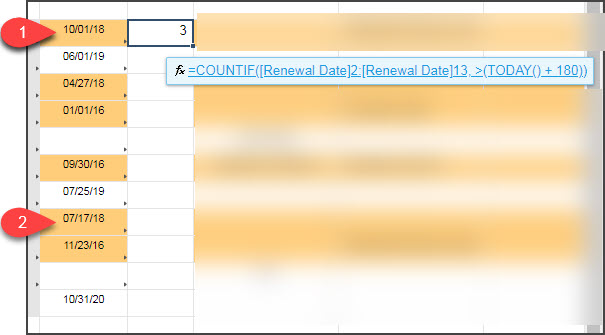# COUNTIF Date is in the next 180 days from today

I'm trying to use the COUNTIF function to search a date column to count how many dates are within the next 180 days from today...but my formulas aren't working correctly.

Failed Attempt 1: =COUNTIF([Renewal Date]2, >=TODAY(+180))

Failed Attempt 2: =COUNTIF([Renewal Date]2, >=TODAY()180)

Failed Attempt 3: =COUNTIF([Renewal Date]2, AND(@cell >= TODAY(), @cell > TODAY(+180)))

What am I doing wrong?

Tags:

• Try this and see if it works:

COUNTIF(Renewal Date1:Renewal Date402, >(TODAY() + 180))

Update the Renewal Date1:Renewal Date 402 with the appropriate first and last cell in the column to be looked at

The formula I am basing this off of is COUNTIF(Finish1:Finish402, >(TODAY() + 10)) which is looking for items in the next 10 days and it works for me.

• This formula isn't calculating correctly.  It's calculating 3, but there are only 2 dates that are within the next 180 days.  See attachment• I think you might actually want all dates that are less than Today + 180... so within the next 180 days.

=COUNTIF(Date1, <=TODAY(180))

This one worked for me. But if you don't want it to count items that are in the past you would also want to edit it to the following.

=COUNTIFS(Date1, <=TODAY(180), Date1, >=TODAY())

• Sorry - edited for your current instance

=COUNTIF([Renewal Date]2, <=TODAY(180))

This one worked for me. But if you don't want it to count items that are in the past you would also want to edit it to the following.

=COUNTIFS([Renewal Date]2, <=TODAY(180), [Renewal Date]2, >=TODAY())

## Help Article Resources

Want to practice working with formulas directly in Smartsheet?

Check out the Formula Handbook template!